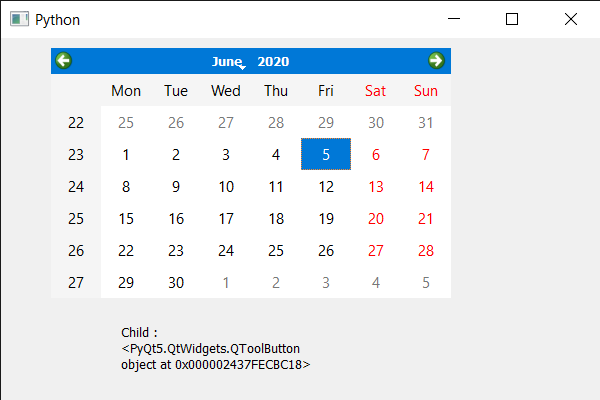# PyQt5 QCalendarWidget – Getting Child of it at given co-ordinate

• Last Updated : 05 Nov, 2021

In this article we will see how we get the child of QCalendarWidget at the given co-ordinate/position. Calendar is not alone a single widget it is a mixture of lots of smaller widget which we refer as calendar child. There are many child such as table view, item delegate etc.

In order to do this we will use childAt method with the QCalendarWidget object.
Syntax : calendar.childAt(x, y)
Argument : It takes two integer as argument
Return : It return child of calendar at given position

Below is the implementation

## Python3

 `# importing libraries``from` `PyQt5.QtWidgets ``import` `*``from` `PyQt5 ``import` `QtCore, QtGui``from` `PyQt5.QtGui ``import` `*``from` `PyQt5.QtCore ``import` `*``import` `sys`  `class` `Window(QMainWindow):` `    ``def` `__init__(``self``):``        ``super``().__init__()` `        ``# setting title``        ``self``.setWindowTitle(``"Python "``)` `        ``# setting geometry``        ``self``.setGeometry(``100``, ``100``, ``600``, ``400``)` `        ``# calling method``        ``self``.UiComponents()` `        ``# showing all the widgets``        ``self``.show()` `    ``# method for components``    ``def` `UiComponents(``self``):` `        ``# creating a QCalendarWidget object``        ``self``.calendar ``=` `QCalendarWidget(``self``)` `        ``# setting geometry to the calendar``        ``self``.calendar.setGeometry(``50``, ``10``, ``400``, ``250``)` `        ``# creating a label``        ``label ``=` `QLabel(``self``)` `        ``# setting geometry``        ``label.setGeometry(``120``, ``280``, ``200``, ``60``)` `        ``# making it multi line``        ``label.setWordWrap(``True``)` `        ``# getting child at position 10, 10``        ``value ``=` `self``.calendar.childAt(``10``, ``10``)` `        ``# setting text to the label``        ``label.setText(``"Child : "` `+` `str``(value))`   `# create pyqt5 app``App ``=` `QApplication(sys.argv)` `# create the instance of our Window``window ``=` `Window()`  `# start the app``sys.exit(App.``exec``())`

Output :My Personal Notes arrow_drop_up